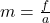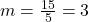## You apply a net force on a soccer ball of 15 N. If the acceleration it has is 5 m/s2 what is the mass of the ball?​

Question

You apply a net force on a soccer ball of 15 N. If the acceleration it has is 5 m/s2 what is the mass of the ball?​

in progress 0
5 months 2021-08-13T01:40:50+00:00 1 Answers 1 views 0

## 3 kg

Explanation:

The mass of the ball can be found by using the formulaf is the force

a is the acceleration

We haveWe have the final answer as

### 3 kg

Hope this helps you